# Income Elasticity of Demand

Income elasticity of demand evaluates the relationship between change in real income of consumers and change in the quantity of the product demanded. It denotes how the number of goods demanded depends upon the change in income of consumers who buy.
• Income elasticity (YED) is defined as the percentage change in demand for an item with a unit percentage change in income.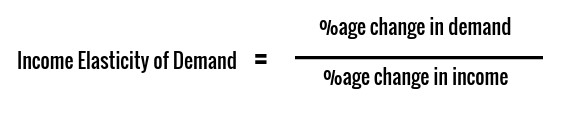#### Example:

• Suppose that the initial monthly income of Charu is Rs.2000 and the quantity demanded of bags by her is 20 units. When her monthly income increases to Rs.3000, the quantity demanded by her also increases to 40 units. Find the income elasticity of demand.
• % change in demand = (40-20)/20 = 100%
• % change in income = (3000 - 2000)/2000 = 50%
• So, Income Elasticity = 100/50 = 2
• Income Elasticity helps to understand the difference between essential items and luxury items. If the income elasticity comes out to be greater than 1, it means it is a luxury item. On the other hand, if income elasticity is less than 1, it means it is an essential item.
• Fashion accessories, cars, holidays, entertainment, air conditioner, etc. are examples of luxury items, and their demand increases at a faster rate as income increases. Income elasticity is more than 1.
• Daily food items, electricity, cooking oil, etc. are examples of essential items and their demand is inelastic with a change in income. Income elasticity is less than 1.
• For inferior items, Income elasticity comes out to be negative. For example, with a rise in income people start eating wheat instead of coarse grains. In this case, the coarse grain is an inferior good whose demand declines with a rise in income.

### Types of Income Elasticity of Demand

• Unitary Income Elasticity – Quantity demanded is proportional to income rise. The value of income elasticity is 1.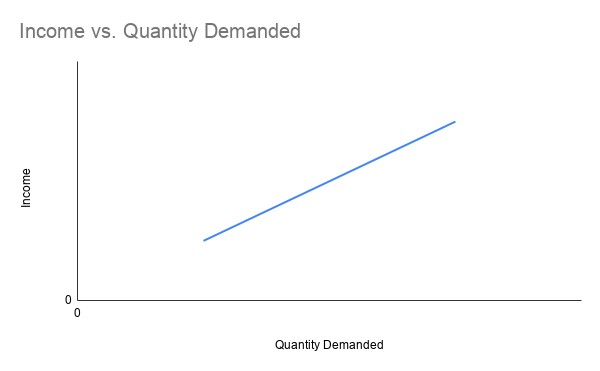• Low-Income Elasticity – Rise in income is lesser than the increase in the quantity demanded. The value of income elasticity is less than 1. Essential items have low-income elasticity.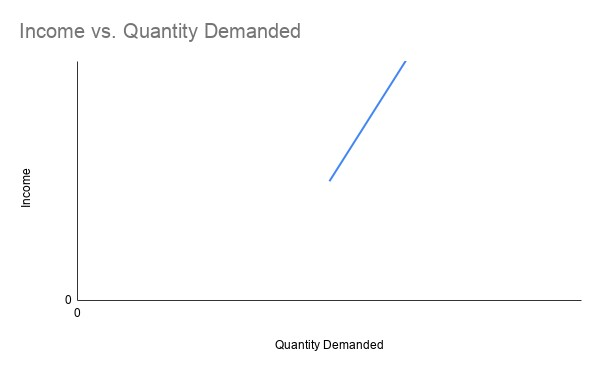• Zero Income Elasticity – The quantity demanded remains the same even if income changes. The value of income elasticity is 0.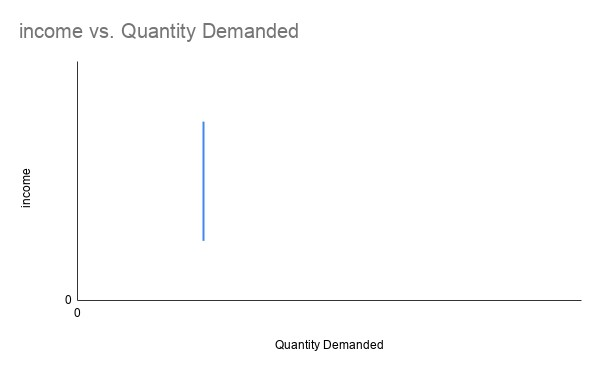• Negative Income Elasticity – An increase in income is followed by a fall in volume demanded. The income elasticity is less than 0. Inferior goods have negative income elasticity.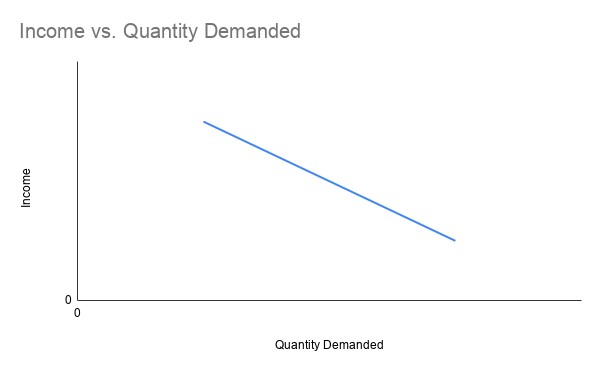• High Income Elasticity – A rise in income is followed by even more significant increases in the quantity demanded. The value of income elasticity is more than 1. Luxury goods have high-income elasticity.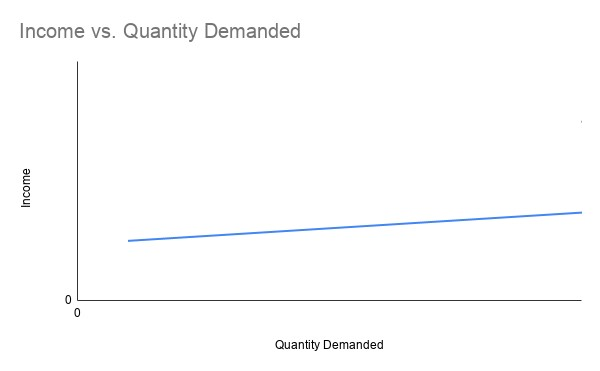## Significance of Income elasticity

• Income elasticity helps us understand the difference between luxury items and necessary items.
• Luxury goods witness high demand when the economy is booming and income level is rising.
• Inferior goods witness decline as income level rises.
• Essential goods are largely unaffected by the income level of a person.

#### Examples:

• The income elasticity of demand is usually strongly positive for
• Fine wines and spirits, high-quality chocolates and luxury holidays overseas.
• Sports cars
• Consumer durables - audiovisual equipment, smart-phones
• Sports and leisure facilities (including gym membership and exclusive sports clubs).
• The income elasticity of demand is lower for
• Staple food products such as bread, vegetables, and frozen foods.
• Mass transport (bus and rail).
• Beer and takeaway pizza.
• Income elasticity of demand is negative (inferior) for cigarettes and urban bus services.